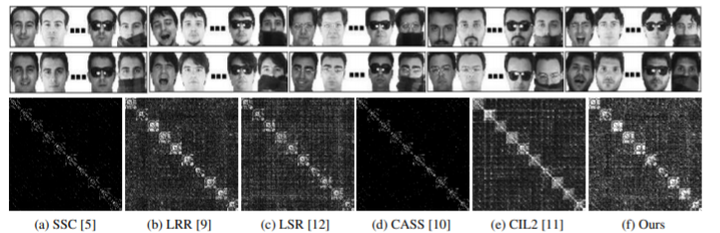# Subspace Clustering by Mixture of Gaussian Regression### Abstract

Subspace clustering is a problem of finding a multisubspace representation that best fits sample points drawn from a high-dimensional space. The existing clustering models generally adopt different norms to describe noise, which is equivalent to assuming that the data are corrupted by specific types of noise. In practice, however, noise is much more complex. So it is inappropriate to simply use a certain norm to model noise. Therefore, we propose Mixture of Gaussian Regression (MoG Regression) for subspace clustering by modeling noise as a Mixture of Gaussians (MoG). The MoG Regression provides an effective way to model a much broader range of noise distributions. As a result, the obtained affinity matrix is better at characterizing the structure of data in real applications. Experimental results on multiple datasets demonstrate that MoG Regression significantly outperforms state-of-the-art subspace clustering methods

Type
Publication
IEEE Conference on Computer Vision and Pattern Recogonition